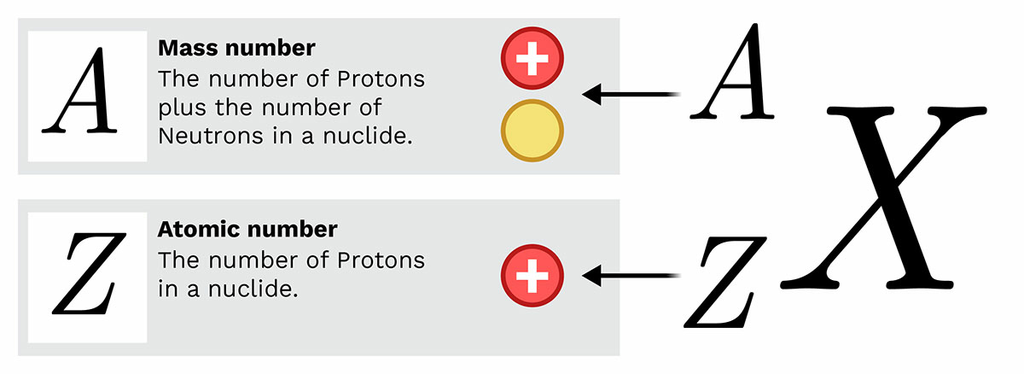In order to properly track what is happening during the different types of radioactive decay, scientists use specific notation to form a decay equation. There are two important numbers in this equation: A - the mass number. This is the number of protons plus the number of neutrons in a nuclide. And Z the atomic number. This is the number of protons in a nuclide.